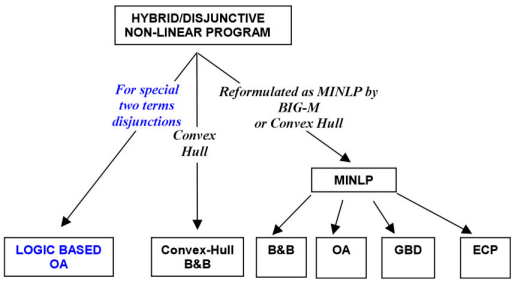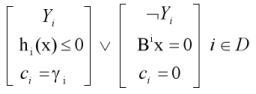# Solution Algorithms for Nonlinear Disjunctive/Hybrid ProgramsFor nonlinear problems only LOGIC BASED OA (Turkay & Grossmann, 1996a) is implemented in LogMIP.
We cannot solve non-linear disjunctive programs with disjunctions containing more than two terms.
The plan is to implement other algorithms in next releases.
Special two terms disjunctions involved in Logic Based OA algorithm, have the following formulation:This special disjunction involves two terms handled by one variable, the first term applies when the Yi is true; the second when it is false. In the second term a subset of variables are set to zero.
The LOGIC BASED OA algorithm was mainly generated to solve the synthesis problem of chemical processes, although it has been applied to examples in other areas.

Synthesis problem example